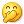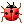# C++11 标准新特性：Defaulted 和 Deleted 函数

IBMdW 发布于 2012/12/26 07:52

Defaulted 函数

C++ 的类有四类特殊成员函数，它们分别是：默认构造函数、析构函数、拷贝构造函数以及拷贝赋值运算符。这些类的特殊成员函数负责创建、初始化、销毁，或者拷贝 类的对象。如果程序员没有显式地为一个类定义某个特殊成员函数，而又需要用到该特殊成员函数时，则编译器会隐式的为这个类生成一个默认的特殊成员函数。例 如：

 ```class X{ private: int a; }; X x;```

 ```class X{ public: X(int i){ a = i; } private: int a; }; X x; // 错误 , 默认构造函数 X::X() 不存在```

 ```class X{ public: X(){}; // 手动定义默认构造函数 X(int i){ a = i; } private: int a; }; X x; // 正确，默认构造函数 X::X() 存在```

Defaulted 函数的提出

 ```class X{ public: X()= default; X(int i){ a = i; } private: int a; }; X x;```

Defaulted 函数定义语法

Defaulted 函数是 C++11 标准引入的函数定义新语法，defaulted 函数定义的语法如图 1 所示：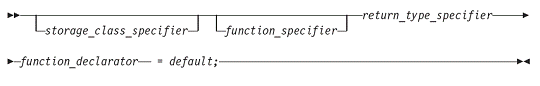Defaulted 函数的用法及示例

Defaulted 函数特性仅适用于类的特殊成员函数，且该特殊成员函数没有默认参数。例如：

 ```class X { public: int f() = default; // 错误 , 函数 f() 非类 X 的特殊成员函数 X(int) = default; // 错误 , 构造函数 X(int, int) 非 X 的特殊成员函数 X(int = 1) = default; // 错误 , 默认构造函数 X(int=1) 含有默认参数 };```

Defaulted 函数既可以在类体里（inline）定义，也可以在类体外（out-of-line）定义。例如：

 ```class X{ public: X() = default; //Inline defaulted 默认构造函数 X(const X&); X& operator = (const X&); ~X() = default; //Inline defaulted 析构函数 }; X::X(const X&) = default; //Out-of-line defaulted 拷贝构造函数 X& X::operator = (const X&) = default; //Out-of-line defaulted // 拷贝赋值操作符```

 ```class X { private: int x; }; class Y: public X { private: int y; }; int main(){ X* x = new Y; delete x; }```

 ```class X { public: virtual ~X(){}; // 手动定义虚析构函数 private: int x; }; class Y: public X { private: int y; }; int main(){ X* x = new Y; delete x; }```

 ```class X { public: virtual ~X()= defaulted; // 编译器自动生成 defaulted 函数定义体 private: int x; }; class Y: public X { private: int y; }; int main(){ X* x = new Y; delete x;```

}

Deleted 函数

 ```class X{ public: X(); }; int main(){ X x1; X x2=x1; // 正确，调用编译器隐式生成的默认拷贝构造函数 X x3; x3=x1; // 正确，调用编译器隐式生成的默认拷贝赋值操作符 }```

Deleted 函数的提出

 ```class X{ public: X(); X(const X&) = delete; // 声明拷贝构造函数为 deleted 函数 X& operator = (const X &) = delete; // 声明拷贝赋值操作符为 deleted 函数 }; int main(){ X x1; X x2=x1; // 错误，拷贝构造函数被禁用 X x3; x3=x1; // 错误，拷贝赋值操作符被禁用 }```

Deleted 函数定义语法

Deleted 函数是 C++11 标准引入的函数定义新语法，deleted 函数定义的语法如图 2 所示：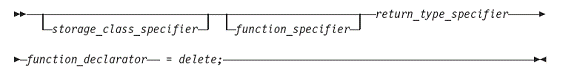Deleted 函数的用法及示例

Deleted 函数特性还可用于禁用类的某些转换构造函数，从而避免不期望的类型转换。在清单 12 中，假设类X只支持参数为双精度浮点数 double 类型的转换构造函数，而不支持参数为整数 int 类型的转换构造函数，则可以将参数为 int 类型的转换构造函数声明为 deleted 函数。

 ```class X{ public: X(double); X(int) = delete; }; int main(){ X x1(1.2); X x2(2); // 错误，参数为整数 int 类型的转换构造函数被禁用 }```

Deleted 函数特性还可以用来禁用某些用户自定义的类的new操作符，从而避免在自由存储区创建类的对象。例如：

 ```#include using namespace std; class X{ public: void *operator new(size_t) = delete; void *operator new[](size_t) = delete; }; int main(){ X *pa = new X; // 错误，new 操作符被禁用 X *pb = new X; // 错误，new[] 操作符被禁用 }```

 ```class X { public: X(const X&); }; X::X(const X&) = delete; // 错误，deleted 函数必须在函数第一次声明处声明```

 ```int add (int,int)=delete; int main(){ int a, b; add(a,b); // 错误，函数 add(int, int) 被禁用 }```

 ```#include using namespace std; int add(int,int) = delete; double add(double a,double b){ return a+b; } int main(){ cout << add(1,3) << endl; // 错误，调用了 deleted 函数 add(int, int) cout << add(1.2,1.3) << endl; return 0; }```

0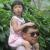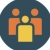Java也一样。如果你定义了一个带参数的构造方法，必须自己定义一个空参数的构造方法，否则当new MyObject(); 时会报错. 只不过Java会编译成字节码而不是本地代码，所以没有这个特性需要
0